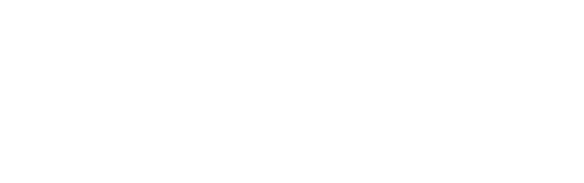# Using Python to calculate northern hemisphere’s surface land coverage

Yesterday during my lunch break I was rather bored; it is unseasonably cold for the fall, even in Calgary, and a bit foggy too.
For something to do I browsed the Earth Science beta on Stack Exchange looking for interesting questions (as an aside, I encourage readers to look at the unanswered questions).
There was one that piqued my curiosity, “In the northern hemisphere only, what percentage of the surface is land?”.
It occurred to me that I could get together an answer using an equal area projection map and a few lines of Python code; and indeed in 15 minutes I whipped-up this workflow:

• Invert and import this B/W image of equal area projection (Peters) for the Northern hemisphere (land = white pixels).Source of original image (full globe): Wikimedia Commons

• Store the image as a Numpy array.
• Calculate the total number of pixels in the image array (black + white).
• Calculate the total number of white pixels (1s) by summing the entire array. Black pixels (0s) will not contribute.
• Calculate percentage of white pixels.

The result I got is 40.44%. Here’s the code:

```# import libraries
import numpy as np
from skimage import io
from matplotlib import pyplot as plt

# import image

# check the image
fig = plt.figure(figsize=(20, 10))
ax.set_xticks([])
ax.set_yticks([])
plt.imshow(north_equal_area, cmap = 'gray');

# Do the calculations
r, c = np.shape(north_equal_area)
sz =  r*c
s = np.sum(north_equal_area)
print(np.round(s/sz*100, decimals=2))
>>> 40.44```

As suggested in a comment to my initial answer, I run the same Python script for the entire globe and got the expected 30% land coverage:

```# import image